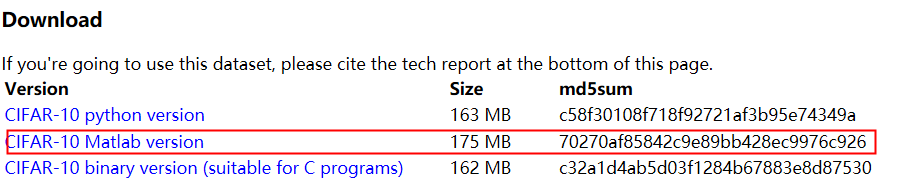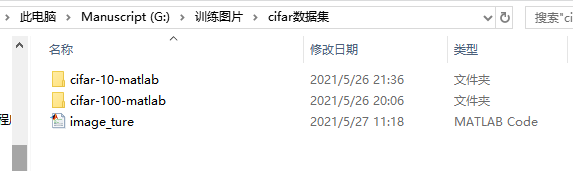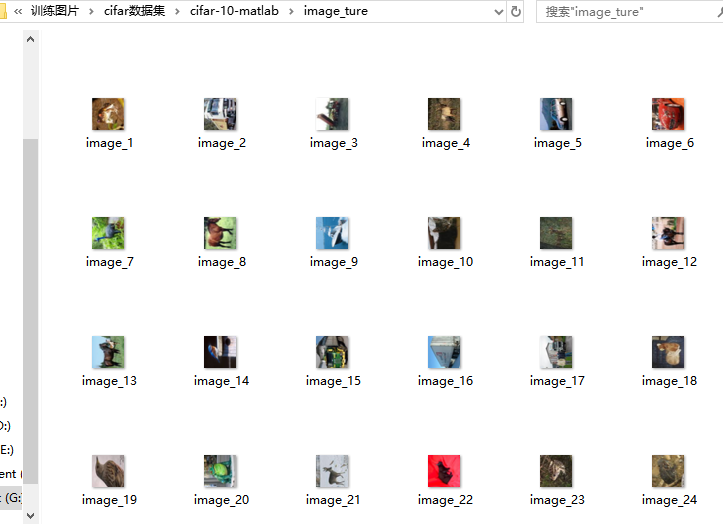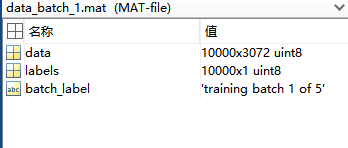# matlab怎么把CIFAR数据集中的图片读取出来``````clear;
close all;
tic;
load('G:\训练图片\cifar-10-matlab\cifar-10-batches-mat\data_batch_1.mat')
image_number=size(labels,1);
imData = uint8(zeros(image_number, 32, 32, 3));  %96个image_number*32的矩阵
dataColor = uint8(zeros(32, 32, 3));      %三个颜色通道
hwait=waitbar(0,'计算中...');
path = 'G:\训练图片\cifar-10-matlab\image_ture/'; % 保存的文件夹位置
prefix = 'image_'; % 文件名前缀
format = 'png'; % （图片）文件格式
suffix = strcat('.',format); % 文件后缀
for i = 1:image_number
value = 100 * i / image_number;
waitbar(i/image_number, hwait, sprintf('计算中:%3.2f%%',value));
data1 = data(i,:);
data1 = data1';
dataColor = reshape(data1, [32, 32, 3]);
imwrite(dataColor,strcat(path, prefix, num2str(i), suffix), format);
imData(i,:,:,:) = dataColor;
end
close(hwait);
toc;
``````cifar数据集是一个用于去噪的训练集，他的官网给出两种文件，分别为“cifar-10-batch-mat和cifar-100=batch-mat。前者有10种类型的图片，后者有100中类别的图片。
cifar-10-batch-mat中有7个文件，其中有五个训练数据和一个测试数据，还有一个标签说明文件（batches.meta）。五个训练数据中每个训练数据是一个大小为10000* 3072（如下图中的data所示）的矩阵，意思表明有10000张图片，每张图片大小为32* 32，一行代表一张图片（大小为：32* 32* 3）。label表示他的标签说明